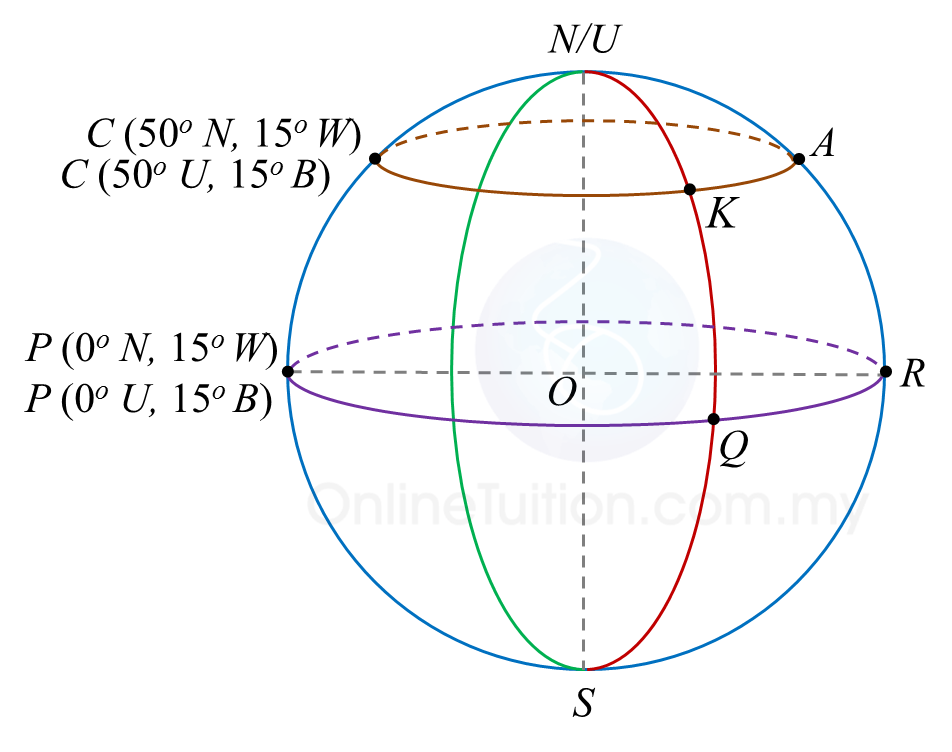# 9.6 SPM Practice (Long Questions)

Question 6:
Diagram below shows the locations of points P, Q, R, A, K and C, on the surface of the earth. O is the centre of the earth.(a) Find the location of A.

(b) Given the distance QR is 3240 nautical miles, find the longitude of Q.

(c) Calculate the distance, in nautical miles of KA, measured along the common parallel latitude.

(d) An aeroplane took off from A and flew due west to K along the common parallel of latitude. Then, it flew due south to Q. The average speed of the aeroplane was 550 knots.
Calculate the total time, in hours, taken for the whole flight.

Solution:
(a)
Longitude of A = (180o – 15o) = 165o E
Latitude of A = 50o N
Therefore, position of A = (50o N, 165o E).

(b)

(c)
Distance of KA
= 54 x 60 x cos 50o
= 2082.6 nautical miles

(d)